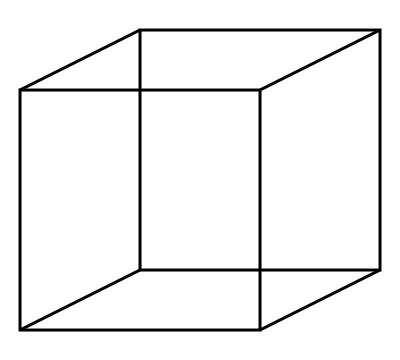# Solve this cube if you can!Positive integers are written on all faces of a cube, one on each. At each corner of the cube, the product of the numbers on the faces that meet at the corner is written. The sum of the numbers written at all the corners is $2004$. If $T$ denotes the sum of the numbers on all faces, find the sum of all possible values of $T$.

×